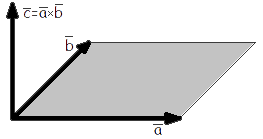Study of mathematics online.
Study math with us and make sure that "Mathematics is easy!"

# Cross product of two vectors

Definition. Cross product (vector product) of vector a by the vector b is the vector c, the length of which is numerically equal to the area of the parallelogram constructed on the vectors a and b, perpendicular to the plane of this vectors and the direction so that the smallest rotation from a to b around the vector c was carried out counter-clockwise when viewed from the terminal point of c (Fig. 1).Fig. 1

## Cross product formulas

Cross product of two vectors a = {ax; ay; az} and b = {bx; by; bz} in Cartesian coordinates is a vector whose value can be calculated using the following formulas:

 a × b = i j k = i(aybz - azby) - j(axbz - azbx) + k(axby - aybx) ax ay az bx by bz
a × b = {aybz - azby; azbx - axbz; axby - aybx}

## Cross product properties

• Geometric interpretation.
The magnitude of the cross product of two vectors a and b is equal to the area of the parallelogram constructed on these vectors:

Ap = |a × b|

• Geometric interpretation.
The area of the triangle constructed on the vectors a and a is equal to half the magnitude of the cross product of this vectors:
 AΔ = 1 |a × b| 2
• Cross product of two non-zero vectors a and b is equal to zero if and only if the vectors are collinear.
• The vector c that is equal to the cross product of non-zero vectors a and b, is perpendicular to these vectors.

c = a × b    =>    c a and c b

• a × b = -b × a
• (k a) × b = a × (k b) = k (a × b)
• (a + b) × c = a × c + b × c

## Cross product examples

Example 1. Find the cross product of a = {1; 2; 3} and b = {2; 1; -2}.

Solution:

 a × b = i j k = 1 2 3 2 1 -2

= i(2 · (-2) - 3 · 1) - j(1 · (-2) - 2 · 3) + k(1 · 1 - 2 · 2) =

= i(-4 - 3) - j(-2 - 6) + k(1 - 4) = -7i + 8j - 3k = {-7; 8; -3}Example 2. Find the area of a triangle formed by vectors a = {-1; 2; -2} and b = {2; 1; -1}.

Solution: Calculate the cross product of these vectors:

 a × b = i j k = -1 2 -2 2 1 -1

= i(2 · (-1) - (-2) · 1) - j((-1) · (-1) - (-2) · 2) + k((-1) · 1 - 2 · 2) =

= i(-2 + 2) - j(1 + 4) + k(-1 - 4) = -5j - 5k = {0; -5; -5}

From the properties of the cross product:

 AΔ = 1 |a × b| = 1 √02 + 52 + 52 = 1 √25 + 25 = 1 √50 = 5√2 2 2 2 2 2